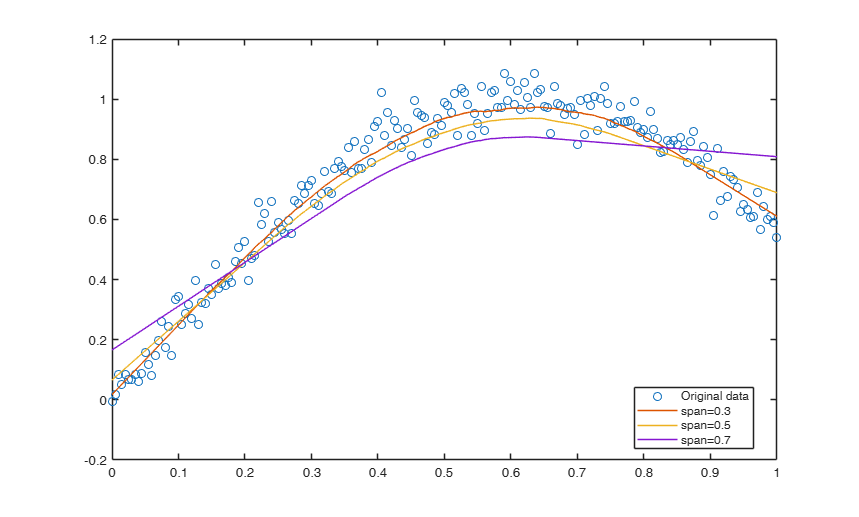# supsmu

supsmu smooths scatterplots using Friedman's supersmoother algorithm.

## Syntax

• smo=supsmu(x,y)example
• smo=supsmu(x,y,Name,Value)example

## Description

This function implements the supersmoother. This is basically the function supsmu written in MALTAB by Douglas M. Schwarz.

Email: dmschwarz=ieee*org, dmschwarz=urgrad*rochester*edu Real_email = regexprep(Email,{'=','*'},{'@','.'}) See the section "More About" of this file for the details of of the modifications which have been made.

 smo =supsmu(x, y) Example of use of supsmu with all the default options.

 smo =supsmu(x, y, Name, Value) Example of use of option Span.

## Examples

expand all

### Example of use of supsmu with all the default options.

x = linspace(0,1,201);
y = sin(2.5*x) + 0.05*randn(1,201);
smo = supsmu(x,y);
plot(x,y,'o',x,smo)

###Example of use of option Span.

x = linspace(0,1,201);
y = sin(2.5*x) + 0.05*randn(1,201);
smo = supsmu(x,y,'Span',0.3);
smo1 = supsmu(x,y,'Span',0.5);
smo2 = supsmu(x,y,'Span',0.7);
plot(x,y,'o',x,[smo; smo1; smo2])
legend({'Original data' 'span=0.3' 'span=0.5' 'span=0.7'},'Location','best')## Input Arguments

### x — x values for smoothing. Vector.

Predictor variable containing the abscissa values specified as a vector of length n, where n is the number of observations.

Data Types: single| double

### y — y values for smoothing. Vector.

Response variable which has to be smoothed, specified as a vector of length n, where n is the number of observations.

Data Types: single| double

### Name-Value Pair Arguments

Specify optional comma-separated pairs of Name,Value arguments. Name is the argument name and Value is the corresponding value. Name must appear inside single quotes (' '). You can specify several name and value pair arguments in any order as  Name1,Value1,...,NameN,ValueN.

Example:  'Weights',1:n , 'Span',0.2 ,

### Weights —weights for the observations.vector.

Row or column vector of positive numbers of length n containing the weights associated to each observations. If w is not specified we assum $w=1$ for $i=1, 2, \ldots, n$.

Example:  'Weights',1:n 

Data Types: double

### Span —fraction of the observations in the span.scalar.

This option sets the width of a fixed-width smoothing operation relative to the number of data points, 0 < Span < 1.

Setting this to be non-zero disables the supersmoother algorithm. Default is 0 (use supersmoother).

Example:  'Span',0.2 

Data Types: double

### Period —Sets the period of periodic data.default is Inf (infinity) which implies that the data is not periodic.

Can also be set to zero for the same effect.

Example - 'Period',1 Data Types - double Alpha Sets a small-span penalty to produce a greater smoothing effect. 0 < Alpha < 10, where 0 does nothing and 10 produces the maximum effect. Default = 0. This paramter controls the smoothness of the fitted curve. Values up to 10 indicate increasing smoothness.

Example - 'Alpha',5 Data Types - double Unsorted Sorted or unsorted data. Boolean.

If the data points are not already sorted in order of the x values then setting this to true will sort them.

Default is false.

Example:

Data Types: double Alpha Sets a small-span penalty to produce a greater smoothing effect. 0 < Alpha < 10, where 0 does nothing and 10 produces the maximum effect. Default = 0. This paramter controls the smoothness of the fitted curve. Values up to 10 indicate increasing smoothness. Example - 'Alpha',5 Data Types - double Unsorted Sorted or unsorted data. Boolean. If the data points are not already sorted in order of the x values then setting this to true will sort them. Default is false. Example - 'Unsorted',5 Data Types - true

## Output Arguments

### smo —smoothed values.  Vector

A vector with the same dimension of y containing smoothed values, that is the y values on the fitted curve.

The supersmoother algorithm computes three separate smooth curves from the input data with symmetric spans of 0.05*n, 0.2*n and 0.5*n, where n is the number of data points. The best of the three smooth curves is chosen for each predicted point using leave-one-out cross validation. The best spans are then smoothed by a fixed-span smoother (span = 0.2*n) and the prediction is computed by linearly interpolating between the three smooth curves. This final smooth curve is then smmothed again with a fixed-span smoother (span = 0.05*n).

According to comments by Friedman, "For small samples (n < 40) or if there are substantial serial correlations between observations close in x-value, then a prespecified fixed span smoother (span > 0) should be used. Reasonable span values are 0.2 to 0.4." This function is basically equal to the function supsmu written in MALTAB by Douglas M. Schwarz.

Email: dmschwarz=ieee*org, dmschwarz=urgrad*rochester*edu Real_email = regexprep(Email,{'=','*'},{'@','.'}) The following modifications with respect to the original function have been made:

 In case of constant values of x over a span program was producng NA. Modifications have been done in the subroutinesto cope with this case.

 The formula for the cross validation residuals in presence of constant values has been introduced.

 All the Mlint suggestions have been incorporated.

 The help has been put inside the FSDA style.

 A series of examples which explore the different options has been added.

Friedman, J. H. (1984). A Variable Span Smoother. Tech. Rep. No. 5, Laboratory for Computational Statistics, Dept. of Statistics, Stanford Univ., California.